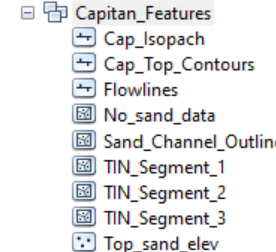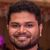# Exporting List of Geodatabase Contents

1128
4
07-17-2020 12:15 PMOccasional Contributor

@Tim Hopper had posted in a previous thread a toolbox for exporting a list which worked great, but I'm hoping someone can help me expand on it.

My client's databases have feature datasets as well as feature classes, and each feature dataset contains different types of features. I have several of these gdbs with related but hopefully not duplicate data from various sources. I need to get a report to management as to what we actually have. The goal would be to consolidate/organize and move it to Pro.

Is there a way to get the hierarchical  structure of a database into a csv file?

For example, here is snippet of a part of one of the databases:In a perfect world, the output would be a csv file that listed the items similar to:

GDB Name, Feature Data Set Name, Feature Class Name, Type of Feature

So for my example,

Cap_Final.gdb, Capitan_Features, Cap_Isopach, Line (Etc)

I also found some code on github that also seemed to get close, but I keep getting errors, and it doesn't export the feature type which would be really handy.

If anyone can give me a hand or help me sort out some python I would appreciate it. I am a beginner at arcpy, so any help would be appreciated.

4 RepliesbyMVP Frequent Contributor
``import arcpyimport osgdb = r'PathToYourGDBhere'arcpy.env.workspace = gdbfcs = arcpy.ListFeatureClasses()print("StandAlone Feature Classes in " + gdb)print("\n")for fc in fcs:      fc_path = os.path.join(gdb, fc)      desc = arcpy.Describe(fc_path)      print("____________")      print(fc)      print(desc.shapeType)      print("____________\n")      print("____________\n")print("____________\n")fds = arcpy.ListDatasets("", "Feature")for fd in fds:    print("\nFeature Classes in feature Dataset - " + fd)    fds_path = os.path.join(gdb, fd)    arcpy.env.workspace = fds_path    fcs = arcpy.ListFeatureClasses()    for fc in fcs:          fc_path = os.path.join(fds_path, fc)          desc = arcpy.Describe(fc_path)          print("____________")          print(fc)          print(desc.shapeType)          print("____________\n")        ‍‍‍‍‍‍‍‍‍‍‍‍‍‍‍‍‍‍‍‍‍‍‍‍‍‍‍‍‍‍‍‍‍‍‍‍‍‍‍‍‍‍‍‍‍‍‍‍‍‍‍‍‍‍‍‍‍‍‍‍‍‍‍‍‍‍‍‍‍‍‍‍‍‍‍‍‍‍‍‍‍``byMVP Frequent Contributor

This is for one GDB, can add a Walk to iterate through GDBs also

``import arcpyimport osgdb = r'C:\Users\DaveP\Documents\ArcGIS\Default.gdb'arcpy.env.workspace = gdbfcs = arcpy.ListFeatureClasses()print("StandAlone Feature Classes in " + gdb)print("\n")for fc in fcs:      fc_path = os.path.join(gdb, fc)      desc = arcpy.Describe(fc_path)      print("____________")      print(fc)      print(desc.shapeType)      print("____________\n")      print("____________\n")print("____________\n")fds = arcpy.ListDatasets("", "Feature")for fd in fds:    print("\nFeature Classes in feature Dataset - " + fd)    fds_path = os.path.join(gdb, fd)    arcpy.env.workspace = fds_path    fcs = arcpy.ListFeatureClasses()    for fc in fcs:          fc_path = os.path.join(fds_path, fc)          desc = arcpy.Describe(fc_path)          print("____________")          print(fc)          print(desc.shapeType)          print("____________\n")        ‍‍‍‍‍‍‍‍‍‍‍‍‍‍‍‍‍‍‍‍‍‍‍‍‍‍‍‍‍‍‍‍‍‍‍‍‍‍‍‍``Occasional Contributor

Thank you, David! I used this, plus some snippets I found in various places, and now I have a listing of everything in the gdb output to a comma-delmited textfile.

I don't know how to share the python like you did, but I've attached the file.

``import arcpyimport osgdb = r'C:\Users\Celeste\TEMP\CapitanAquiferFinal.gdb'textFile = r'C:\Users\Celeste\TEMP\test9.txt'f = open(textFile, "a")arcpy.env.workspace = gdbfcs = arcpy.ListFeatureClasses()for fc in fcs:      fc_path = os.path.join(gdb, fc)      desc = arcpy.Describe(fc_path)      f.write(gdb + ","  + "stand-alone" + fc + "\n")      fds = arcpy.ListDatasets("", "Feature")for fd in fds:        fds_path = os.path.join(gdb, fd)    arcpy.env.workspace = fds_path    fcs = arcpy.ListFeatureClasses()    for fc in fcs:          fc_path = os.path.join(fds_path, fc)          desc = arcpy.Describe(fc_path)          f.write(gdb + "," + fd + "," + fc + "," + desc.shapeType + "\n")gdb = r'C:\Users\Celeste\TEMP\CapitanAquiferFinal.gdb'arcpy.env.workspace = gdbfrs = arcpy.ListRasters("*","*")for fs in frs:    fs_path = os.path.join(gdb, fs)    desc = arcpy.Describe(fs_path)    f.write(gdb + ","  + "grids," + fs + "," + desc.format + "\n")f.close()‍‍‍‍‍‍‍‍‍‍‍‍‍‍‍‍‍‍‍‍‍‍‍‍‍‍‍‍‍‍‍‍‍‍‍‍‍‍‍‍‍‍‍‍‍‍‍``byMVP Regular Contributor

Updated your post to include the Python code.